## Tried and Tested Tips to Run & Interpret One-Way ANOVA Test in SPSS

One-way analysis of variance (ANOVA) checks the existence of a statistically significant difference among two or more independent groups. It uses the variance to determine if the means are equal or not. ANOVA gives insights to two groups that are statistically similar or different.

R.A. Fisher developed this technique which is referred to as Fisher's ANOVA, too. It is comparative while applying to strategies such as T-test and Z-test to check and compare the mean & the variance of two or more independent groups. Notwithstanding, to check the difference ANOVA is best connected where multiple tests are required to perform.

The use of this parametric strategy includes certain  assumptions:

1. Independence of Case: the sample should be randomly selected. All the samples should be independent of each other.
2. Normality: the samples should be normally distributed. Either of them can be used to determine the normality of the group or sample - Kolmogorov-Smirnov  the Shapiro-Wilk

3. Outliers: There should be no outliers. If at all they are present in the sample, we need to treat them before performing the final ANOVA test.

4. Homogeneity: This means that the variance among the groups should be the same. We can apply Levene's test to check the homogeneity in the samples.

Flow chart of ANOVA in SPSS: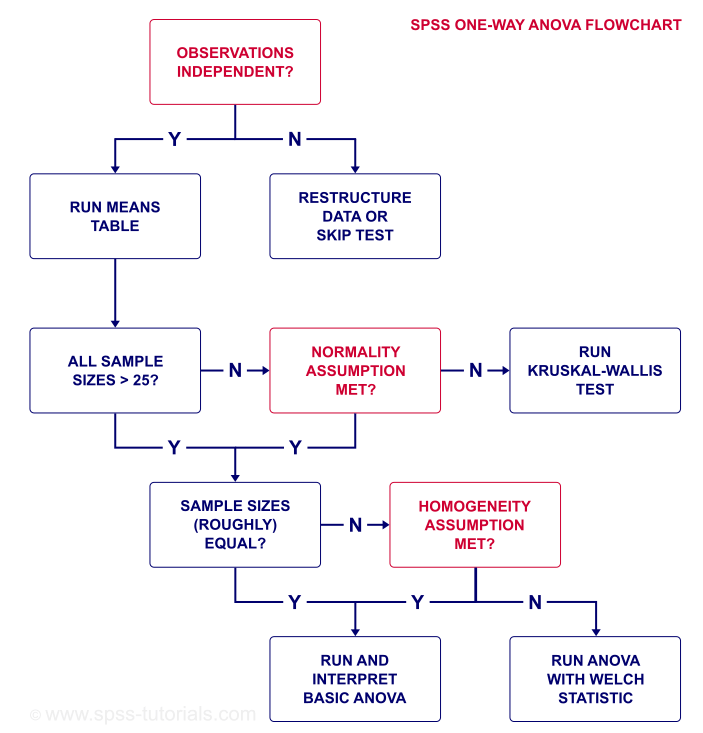## HYPOTHESIS TESTING

The hypothesis In a one-way ANOVA are :

Null hypothesis (H0) : is that there is no difference among the groups

Alternative hypothesis (H1) : is that there is a statistically difference between the means and groups.

EXAMPLE: A specialist is keen on seeing if the force of electric stun will influence the time required to tackle a lot of troublesome issues. Eighteen subjects are arbitrarily relegated to the three test states of low stun, medium stun, and high stun. The all out time (in minutes) required to tackle every one of the issues is the measure recorded for each subject.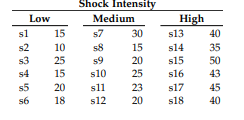STEP 1:  Click on analyse from the menu  bar, then click on one way ANOVA, after clicking ANOVA window will get open.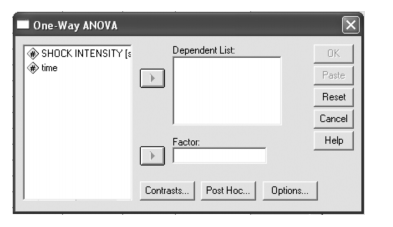STEP 2: Now in the window transfer the dependent variable and factor respectively from the first field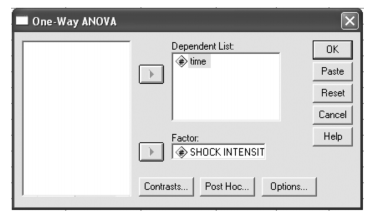STEP 3:  In order to obtain multiple comparisons among all the three shocks levels, we need to select on post hoc analysis and then by selecting scheffe under equal variance window.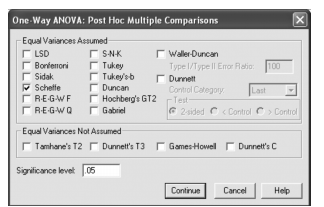STEP 4:  In ANOVA window click to select the option window and then click on descriptive cell to get the descriptive statistics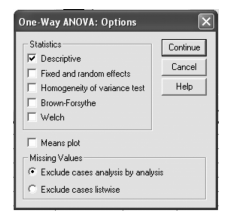STEP 5: Run the analysis by clicking on the OK button.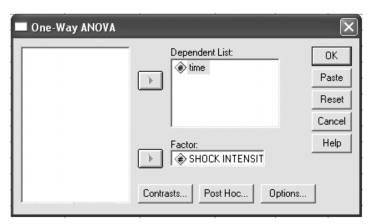CONCLUSIONS AND INTERPRETATIONS :

The table shown below represents the descriptives statistics with mean, standard deviation, standard error, with confidence interval with lower and upper bound, with maximum & minimum for all the variables.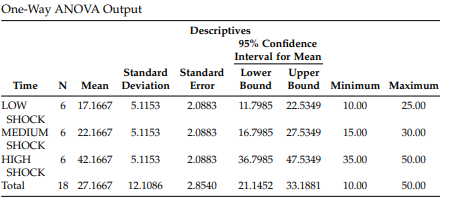The table below shows the f test statistics for the two sample: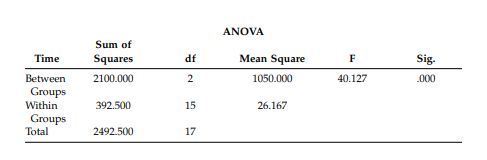The outcomes show that the power of the electric shock significantly affects the time taken to take care of the issues

F(2,15) = 40.127

P value < .001.

The mean qualities for the three shock levels demonstrate that as the shock level expanded from low -> medium-> high intensity, the shock increased simultaneously.